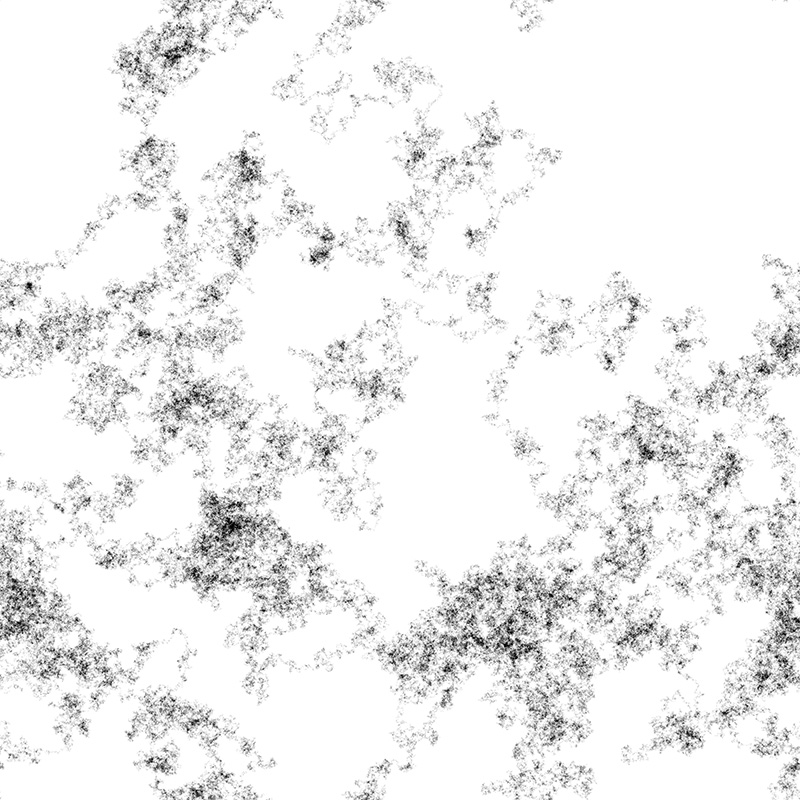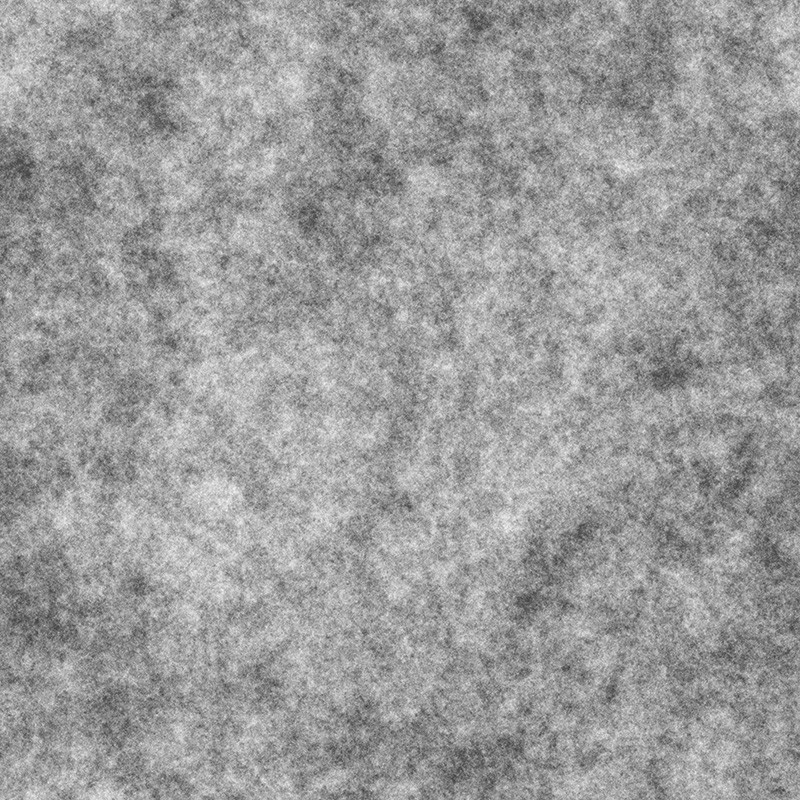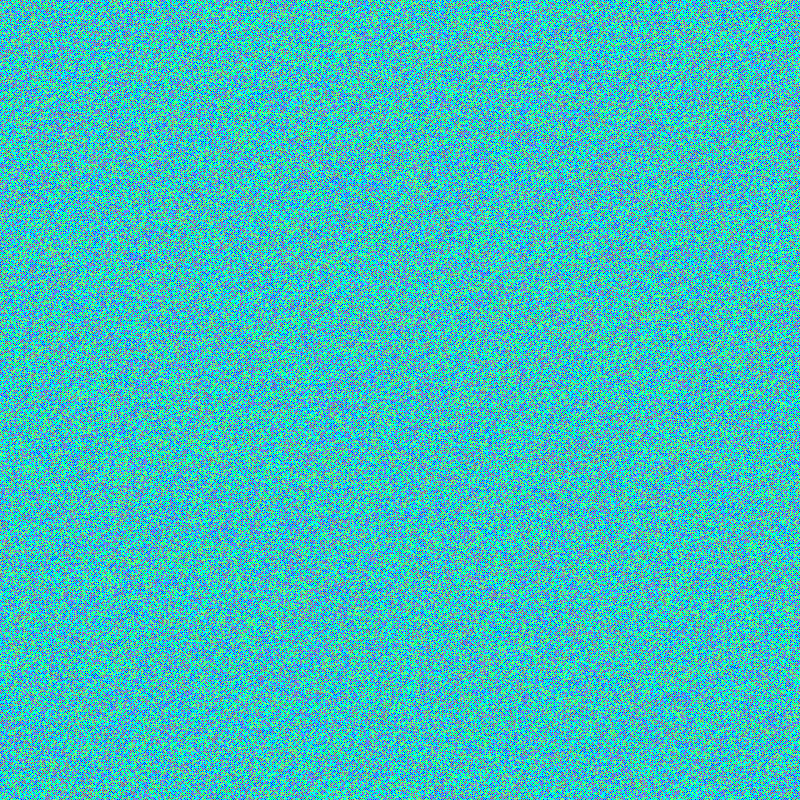# Random walk

Written by Paul Bourke
December 2014

The so called random walk involves a particle that moves in any direction with a uniform random distribution. When implemented by computer to form an image, on each iteration the point can move in one of 8 directions: the 4 cardinal directions and the 4 diagonals, each with equal probability. The following illustrates the nature of the images formed, it is by no means a technical or theoretical treatment but intended to give the reader a sense of the images formed. In the computer graphics domain these are often used to provide a dirty appearance to textured objects. Random walk is proposed as a first approximation to a number of physical process, such a molecular motion, and for some human driven processes such as the stock exchange fluctuations.

In this first example the number of iterations is low such that large portions of the plane are not covered. In what follows, the images are greyscale where the level is given by the number of times the particle passes through that pixel.100 million iterations on a 10,000 x 10,000 grid with periodic bounds.

The following is an order of magnitude more iterations covering almost all pixels at least once. The limit to infinity is a uniform density.1000 million iterations on a 10,000 x 10,000 grid with periodic bounds.

It is quite a good way to reveal how good a random number generator is, for example the following is using the (poor) rand() function, the diagonal structure is obvious for over 2000 million points.5000 million iterations using the rand() function.

The following uses the much better drand48() function. The limit for this discrete random walk on a square lattice as the plane resolution increases is Brownian motion.5000 million iterations using the drand48() function.

Since the direction is randomly chosen on each iteration from a uniform distribution, if one accumulates the direction vector at each pixel the result should be a random field.

The following is a zoom in of the accumulated direction vectors coloured from red to blue to red mapped as a circular colour map from -pi to pi.10,000 million iterations.